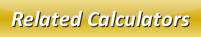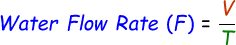# Water Flow Rate Calculator

With the help of our online water flow rate calculator, you will be able to find the amount of water flowing through a pipe or an orifice onto a container per second. This can be done when you know the amount of water collected in the container after t amount of time.

Formula to calculate water flow rate is given below:where,
F = Water flow rate [liters/sec]
V = Water collected in container [liters]
T = Time taken [secs]

Enter the given input values in the below calculator and click the calculate button to find the water flow rate.

 Water Collected in Container (V): [liters] Time Taken (T): [secs] Water Flow Rate (F): [liters/sec]

Latest Calculator Release

Average Acceleration Calculator

Average acceleration is the object's change in speed for a specific given time period. ...

Free Fall Calculator

When an object falls into the ground due to planet's own gravitational force is known a...

Torque Calculator

Torque is nothing but a rotational force. In other words, the amount of force applied t...

Average Force Calculator

Average force can be explained as the amount of force exerted by the body moving at giv...

Angular Displacement Calculator

Angular displacement is the angle at which an object moves on a circular path. It is de...